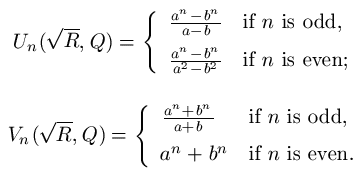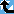# Lehmer primitive part

The Prime Pages keeps a list of the 5000 largest known primes, plus a few each of certain selected archivable forms and classes. These forms are defined in this collection's home page.

Lehmer defined a generalization of Lucas sequences as follows:where a and b are the zeros of the polynomial z2-R1/2z+Q for non-zero rational integers R, Q, and R-4Q

A primitive divisor of a Lehmer is one that does not divide any previous term, and does not divide the product R(R-4Q).  Many of the references below (culminating in [BHV2002]) show that all but a finite number of the terms in a Lehmer sequence have primitive divisors.

If we let n=3rms, where m > 3 is prime and min(r,s)>0. Then the numbers

(V(P,1,n/3)+1)/(V(P,1,n/(3m)+1)
(V(P,1,n/3)-1)/(V(P,1,n/(3m)-1)
are Lehmer primitive parts, with R=P+2 and Q=1, and their product is the Lucas primitive part primU(P,1,n).

###Record Primes of this Type

rankprime digitswhowhencomment
1(V(60145, 1, 7317) - 1)/(V(60145, 1, 27) - 1) 34841 x45 Aug 2019 Lehmer primitive part
2(V(28138, 1, 7587) - 1)/(V(28138, 1, 27) - 1) 33637 x45 Aug 2019 Lehmer primitive part
3(V(48395, 1, 6921) - 1)/(V(48395, 1, 9) - 1) 32382 x45 Aug 2019 Lehmer primitive part
4(V(77786, 1, 6453) + 1)/(V(77786, 1, 27) + 1) 31429 x25 Dec 2012 Lehmer primitive part
5(V(73570, 1, 6309) - 1)/(V(73570, 1, 9) - 1) 30661 x25 Jun 2016 Lehmer primitive part
6(V(28286, 1, 6309) + 1)/(V(28286, 1, 9) + 1) 28045 x25 Jun 2016 Lehmer primitive part
7(V(59936, 1, 4863) + 1)/(V(59936, 1, 3) + 1) 23220 x25 Jan 2013 Lehmer primitive part
8(V(45366, 1, 4857) + 1)/(V(45366, 1, 3) + 1) 22604 x25 Feb 2013 Lehmer primitive part
9(V(23354, 1, 4869) - 1)/(V(23354, 1, 9) - 1) 21231 x25 Feb 2013 Lehmer primitive part
10(V(428, 1, 8019) - 1)/(V(428, 1, 729) - 1) 19184 E1 Jun 2022 Lehmer primitive part, ECPP
11(U(162, 1, 8581) + U(162, 1, 8580))/(U(162, 1, 66) + U(162, 1, 65)) 18814 E1 Jun 2022 Lehmer primitive part, ECPP
12(U(859, 1, 6385) - U(859, 1, 6384))/(U(859, 1, 57) - U(859, 1, 56)) 18567 E1 Jun 2022 Lehmer primitive part, ECPP
13(V(46662, 1, 3879) - 1)/(V(46662, 1, 9) - 1) 18069 x25 Dec 2012 Lehmer primitive part
14(V(447, 1, 6723) + 1)/(V(447, 1, 81) + 1) 17604 E1 Jun 2022 Lehmer primitive part, ECPP
15(V(561, 1, 6309) + 1)/(V(561, 1, 9) + 1) 17319 x25 May 2016 Lehmer primitive part
16(V(1578, 1, 5589) + 1)/(V(1578, 1, 243) + 1) 17098 E1 Jun 2022 Lehmer primitive part, ECPP
17(V(1240, 1, 5589) - 1)/(V(1240, 1, 243) - 1) 16538 E1 Jun 2022 Lehmer primitive part, ECPP
18(U(800, 1, 5725) - U(800, 1, 5724))/(U(800, 1, 54) - U(800, 1, 53)) 16464 E1 Jun 2022 Lehmer primitive part, ECPP
19(V(21151, 1, 3777) - 1)/(V(21151, 1, 3) - 1) 16324 x25 May 2011 Lehmer primitive part
20(U(9275, 1, 3961) + U(9275, 1, 3960))/(U(9275, 1, 45) + U(9275, 1, 44)) 15537 x38 May 2009 Lehmer primitive part

###References

BHV2002
Bilu, Yu., Hanrot, G. and Voutier, P. M., "Existence of primitive divisors of Lucas and Lehmer numbers," J. Reine Angew. Math., 539 (2001) 75--122.  With an appendix by M. Mignotte.  MR1863855 (Annotation available)
Schinzel1963
Schinzel, A., "On primitive prime factors of Lehmer numbers. II," Acta. Arith., 8 (1962/1963) 251--257.  MR 27:1409
Schinzel1968
Schinzel, A., "On primitive prime factors of Lehmer numbers. III," Acta Arith., 15 (1968) 49--70.  MR0232744
Schinzel1970
Schinzel, A., "Corrigendum to the papers "On two theorems of Gelfond and some of their applications" and "On primitive prime factors of Lehmer numbers. III"," Acta Arith., 16 (1969/1970) 101.  MR0246840
Stewart1976
Stewart, C. L., Primitive divisors of Lucas and Lehmer numbers.  In "Transcendence theory: advances and applications (Proc. Conf., Univ. Cambridge, Cambridge, 1976)," Academic Press, London, 1977.  pp. 79--92, MR0476628
Stewart1977b
Stewart, C. L., Primitive divisors of Lucas and Lehmer numbers.  In "Transcendence theory: advances and applications (Proc. Conf., Univ. Cambridge, Cambridge, 1976)," Academic Press, 1977.  London, pp. 79--92, MR 57:16187
Stewart1983
Stewart, C. L., "On divisors of Fermat, Fibonacci, Lucas and Lehmer numbers. III," J. London Math. Soc. (2), 28:2 (1983) 211--217.  MR 85g:11021
Voutier1995
Voutier, P. M., "Primitive divisors of Lucas and Lehmer sequences," Math. Comp., 64:210 (1995) 869--888.  MR1284673 (Annotation available)
Voutier1996
Voutier, P. M., "Primitive divisors of Lucas and Lehmer sequences. II," J. Th\'eor. Nombres Bordeaux, 8:2 (1996) 251--274.  MR1438469
Voutier1998
Voutier, P. M., "Primitive divisors of Lucas and Lehmer sequences. III," Math. Proc. Cambridge Philos. Soc., 123:3 (1998) 407--419.  MR1607969 [From the review: "The main result of this paper is that for any integer n>30 030, the nth element of any Lucas or Lehmer sequence has a primitive divisor."]